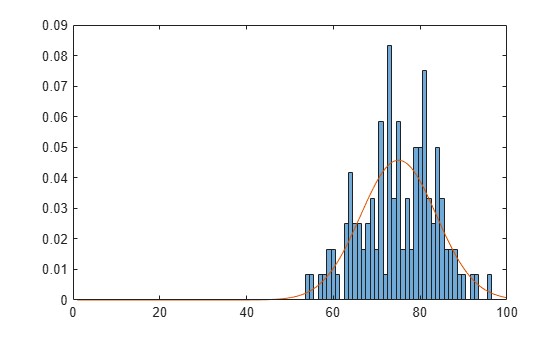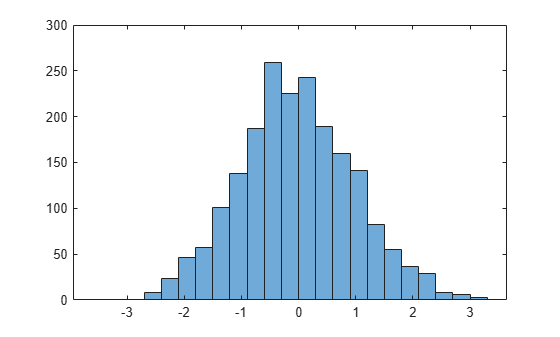## Working with Probability Distributions

Probability distributions are theoretical distributions based on assumptions about a source population. The distributions assign probability to the event that a random variable has a specific, discrete value, or falls within a specified range of continuous values.

Statistics and Machine Learning Toolbox™ offers several ways to work with probability distributions.

For a list of distributions supported by Statistics and Machine Learning Toolbox, see Supported Distributions.

### Probability Distribution Objects

Probability distribution objects allow you to fit a probability distribution to sample data, or define a distribution by specifying parameter values. You can then perform a variety of analyses on the distribution object.

#### Create Probability Distribution Objects

Estimate probability distribution parameters from sample data by fitting a probability distribution object to the data using `fitdist`. You can fit a single specified parametric or nonparametric distribution to the sample data. You can also fit multiple distributions of the same type to the sample data based on grouping variables. For most distributions, `fitdist` uses maximum likelihood estimation (MLE) to estimate the distribution parameters from the sample data. For more information and additional syntax options, see `fitdist`.

Alternatively, you can create a probability distribution object with specified parameter values using `makedist`.

#### Work with Probability Distribution Objects

Once you create a probability distribution object, you can use object functions to:

#### Save a Probability Distribution Object

To save your probability distribution object to a .MAT file:

• In the toolbar, click . This option saves all of the variables in your workspace, including any probability distribution objects.

• In the workspace browser, right-click the probability distribution object and select Save as. This option saves only the selected probability distribution object, not the other variables in your workspace.

Alternatively, you can save a probability distribution object directly from the command line by using the `save` function. `save` enables you to choose a file name and specify the probability distribution object you want to save. If you do not specify an object (or other variable), MATLAB® saves all of the variables in your workspace, including any probability distribution objects, to the specified file name. For more information and additional syntax options, see `save`.

#### Analyze Distribution Using Probability Distribution Objects

This example shows how to use probability distribution objects to perform a multistep analysis on a fitted distribution.

The analysis illustrates how to:

• Fit a probability distribution to sample data that contains exam grades of 120 students by using `fitdist`.

• Compute the mean of the exam grades by using `mean`.

• Plot a histogram of the exam grade data, overlaid with a plot of the pdf of the fitted distribution, by using `plot` and `pdf`.

• Compute the boundary for the top 10 percent of student grades by using `icdf`.

• Save the fitted probability distribution object by using `save`.

`load examgrades`

The sample data contains a 120-by-5 matrix of exam grades. The exams are scored on a scale of 0 to 100.

Create a vector containing the first column of exam grade data.

`x = grades(:,1);`

Fit a normal distribution to the sample data by using `fitdist` to create a probability distribution object.

`pd = fitdist(x,'Normal')`
```pd = NormalDistribution Normal distribution mu = 75.0083 [73.4321, 76.5846] sigma = 8.7202 [7.7391, 9.98843] ```

`fitdist` returns a probability distribution object, `pd`, of the type `NormalDistribution`. This object contains the estimated parameter values, `mu` and `sigma`, for the fitted normal distribution. The intervals next to the parameter estimates are the 95% confidence intervals for the distribution parameters.

Compute the mean of the students' exam grades using the fitted distribution object, `pd`.

`m = mean(pd)`
```m = 75.0083 ```

The mean of the exam grades is equal to the `mu` parameter estimated by `fitdist`.

Plot a histogram of the exam grades. Overlay a plot of the fitted pdf to visually compare the fitted normal distribution with the actual exam grades.

```x_pdf = [1:0.1:100]; y = pdf(pd,x_pdf); figure histogram(x,'Normalization','pdf') line(x_pdf,y)```The pdf of the fitted distribution follows the same shape as the histogram of the exam grades.

Determine the boundary for the upper 10 percent of student exam grades by using the inverse cumulative distribution function (`icdf`). This boundary is equivalent to the value at which the cdf of the probability distribution is equal to 0.9. In other words, 90 percent of the exam grades are less than or equal to the boundary value.

`A = icdf(pd,0.9)`
```A = 86.1837 ```

Based on the fitted distribution, 10 percent of students received an exam grade greater than 86.1837. Equivalently, 90 percent of students received an exam grade less than or equal to 86.1837.

Save the fitted probability distribution, `pd`, as a file named `myobject.mat`.

`save('myobject.mat','pd')`

### Apps and Interactive User Interfaces

Apps and user interfaces provide an interactive approach to working with parametric and nonparametric probability distributions.

• Use the Distribution Fitter app to interactively fit a distribution to sample data, and export a probability distribution object to the workspace.

• Use the Probability Distribution Function user interface to visually explore the effect on the pdf and cdf of changing the distribution parameter values.

• Use the Random Number Generation user interface (`randtool`) to interactively generate random numbers from a probability distribution with specified parameter values and export them to the workspace.

#### Distribution Fitter App

The Distribution Fitter app allows you to interactively fit a probability distribution to your data. You can display different types of plots, compute confidence bounds, and evaluate the fit of the data. You can also exclude data from the fit. You can save the data, and export the fit to your workspace as a probability distribution object to perform further analysis.

Load the Distribution Fitter app from the Apps tab, or by entering `distributionFitter` in the command window. For more information, see Model Data Using the Distribution Fitter App.#### Probability Distribution Function Tool

The Probability Distribution Function user interface visually explores probability distributions. You can load the Probability Distribution Function user interface by entering `disttool` in the command window.#### Random Number Generation Tool

The Random Number Generation user interface generates random data from a specified distribution and exports the results to your workspace. You can use this tool to explore the effects of changing parameters and sample size on the distributions.

The Random Number Generation user interface allows you to set parameter values for the distribution and change their lower and upper bounds; draw another sample from the same distribution, using the same size and parameters; and export the current random sample to your workspace for use in further analysis. A dialog box enables you to provide a name for the sample.### Distribution-Specific Functions and Generic Distribution Functions

Using distribution-specific functions and generic distribution functions is useful for generating random numbers, computing summary statistics inside a loop or script, and passing a cdf or pdf as a function handle to another function. You can also use these functions to perform computations on arrays of parameter values rather than a single set of parameters.

• Distribution-specific functions — Some of the supported distributions have distribution-specific functions. These functions use the following abbreviations, as in `normpdf`, `normcdf`, `norminv`, `normstat`, `normfit`, `normlike`, and `normrnd`:

• `pdf` — Probability density functions

• `cdf` — Cumulative distribution functions

• `inv` — Inverse cumulative distribution functions

• `stat` — Distribution statistics functions

• `fit` — Distribution Fitter functions

• `like` — Negative loglikelihood functions

• `rnd` — Random number generators

• Generic distribution functions — Use `cdf`, `icdf`, `mle`, `pdf`, and `random` with a specified distribution name and parameters.

#### Analyze Distribution Using Distribution-Specific Functions

This example shows how to use distribution-specific functions to perform a multistep analysis on a fitted distribution.

The analysis illustrates how to:

• Fit a probability distribution to sample data that contains exam grades of 120 students by using `normfit`.

• Plot a histogram of the exam grade data, overlaid with a plot of the pdf of the fitted distribution, by using `plot` and `normpdf`.

• Compute the boundary for the top 10 percent of student grades by using `norminv`.

• Save the estimated distribution parameters by using `save`.

You can perform the same analysis using a probability distribution object. See Analyze Distribution Using Probability Distribution Objects.

`load examgrades`

The sample data contains a 120-by-5 matrix of exam grades. The exams are scored on a scale of 0 to 100.

Create a vector containing the first column of exam grade data.

`x = grades(:,1);`

Fit a normal distribution to the sample data by using `normfit`.

`[mu,sigma,muCI,sigmaCI] = normfit(x)`
```mu = 75.0083 ```
```sigma = 8.7202 ```
```muCI = 2×1 73.4321 76.5846 ```
```sigmaCI = 2×1 7.7391 9.9884 ```

The `normfit` function returns the estimates of normal distribution parameters and the 95% confidence intervals for the parameter estimates.

Plot a histogram of the exam grades. Overlay a plot of the fitted pdf to visually compare the fitted normal distribution with the actual exam grades.

```x_pdf = [1:0.1:100]; y = normpdf(x_pdf,mu,sigma); figure histogram(x,'Normalization','pdf') line(x_pdf,y)```The pdf of the fitted distribution follows the same shape as the histogram of the exam grades.

Determine the boundary for the upper 10 percent of student exam grades by using the normal inverse cumulative distribution function. This boundary is equivalent to the value at which the cdf of the probability distribution is equal to 0.9. In other words, 90 percent of the exam grades are less than or equal to the boundary value.

`A = norminv(0.9,mu,sigma)`
```A = 86.1837 ```

Based on the fitted distribution, 10 percent of students received an exam grade greater than 86.1837. Equivalently, 90 percent of students received an exam grade less than or equal to 86.1837.

Save the estimated distribution parameters as a file named `myparameter.mat`.

`save('myparameter.mat','mu','sigma')`

#### Use Probability Distribution Functions as Function Handle

This example shows how to use the probability distribution function `normcdf` as a function handle in the chi-square goodness of fit test (`chi2gof`).

This example tests the null hypothesis that the sample data contained in the input vector, `x`, comes from a normal distribution with parameters µ and σ equal to the mean (`mean`) and standard deviation (`std`) of the sample data, respectively.

```rng('default') % For reproducibility x = normrnd(50,5,100,1); h = chi2gof(x,'cdf',{@normcdf,mean(x),std(x)})```
```h = 0 ```

The returned result `h = 0` indicates that `chi2gof` does not reject the null hypothesis at the default 5% significance level.

This next example illustrates how to use probability distribution functions as a function handle in the slice sampler (`slicesample`). The example uses `normpdf` to generate a random sample of 2,000 values from a standard normal distribution, and plots a histogram of the resulting values.

```rng('default') % For reproducibility x = slicesample(1,2000,'pdf',@normpdf,'thin',5,'burnin',1000); histogram(x)```The histogram shows that, when using `normpdf`, the resulting random sample has a standard normal distribution.

If you pass the probability distribution function for the exponential distribution pdf (`exppdf`) as a function handle instead of `normpdf`, then `slicesample` generates the 2,000 random samples from an exponential distribution with a default parameter value of µ equal to 1.

```rng('default') % For reproducibility x = slicesample(1,2000,'pdf',@exppdf,'thin',5,'burnin',1000); histogram(x)```The histogram shows that the resulting random sample when using `exppdf` has an exponential distribution.